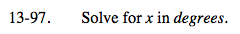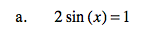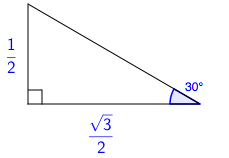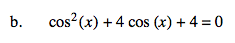### Home > A2C > Chapter Ch13 > Lesson 13.2.1 > Problem13-97

13-97.
1. Solve for x in degrees. Homework Help ✎

1. 2sin(x) = 1

2. cos2(x) + 4cos(x) + 4 = 0Draw a 30°-60°-90° triangle and label the sides.In the first quadrant, the side opposite the 30° is one-half.

In the second quadrant, the angle with sine equal to one-half is 180° − 30° or 150°.

x = 30° + 360°n or x = 150° + 360°nFactor the equation and simplify.

There is no solution.